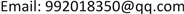1. 前言

2. 利用特征多项式求最小多项式

m A ( λ ) = ( λ − λ 1 ) d 1 ( λ − λ 2 ) d 2 ⋯ ( λ − λ s ) d s , 其中 d i ≤ n i ( i = 1 , 2 , … , s ) .

f A ( λ ) = | λ E − A | = ( λ − 1 ) ( λ − 2 ) 2 ,

m 1 ( λ ) = ( λ − 1 ) ( λ − 2 ) ， ，

m 1 ( A ) = ( A − E ) ( A − 2 E ) = O ，

m A ( λ ) = ( λ − 1 ) ( λ − 2 ) .

3. 最小多项式的应用3.1. 化简任意矩阵多项式

f A ( λ ) = ( λ − 2 ) 3 ,

m 1 ( λ ) = ( λ − 2 ) ， m 2 ( λ ) = ( λ − 2 ) 2 ， m 3 ( λ ) = ( λ − 2 ) 3 ，

m 1 ( A ) = ( A − 2 E ) ≠ O ， m 2 ( A ) = ( A − 2 E ) 2 = O ，

m A ( λ ) = ( λ − 2 ) 2 ，

f ( λ ) = q ( λ ) m A ( λ ) + r ( λ ) ，

f ( A ) = r ( A ) = 330 A − 539 E = [ 121 0 0 330 − 209 330 330 − 330 451 ] 。

3.2. 求线性空间的维数和一组基

1) W 的维数等于 m A ( λ ) 的次数 k ，即 dim ( W ) = ∂ ( m A ( λ ) ) = k ；

2) E , A , A 2 , ⋯ , A k − 1 为 W 的一组。

f A ( λ ) = ( λ − 1 ) ( λ − w ) ( λ − w 2 ) ，

w 2 = − 1 − 3 i 2 ， w 3 = 1 ，

m A ( λ ) = f A ( λ ) = ( λ − 1 ) ( λ − w ) ( λ − w 2 ) ，

dim ( W ) = ∂ ( m A ( λ ) ) = 3 ，

3.3. 求解矩阵方程

( m A ( λ ) , m B ( λ ) ) = 1 ，

X = ( A ˜ − B ˜ ) − 1 C = − ∑ k = 0 r − 1 ∑ s = 0 k α r − s A r − 1 − k C B k − s u ( B ) ，

m A ( λ ) u ( λ ) + m B ( λ ) v ( λ ) = 1 。

A = [ 1 1 0 − 1 − 1 1 − 2 − 2 0 ] ， B = [ 0 1 0 0 0 1 − 1 − 2 − 1 ] ， C = [ 1 0 1 0 0 0 1 0 1 ] ，

m A ( λ ) = λ 3 + 2 λ ， m B ( λ ) = λ 3 + λ 2 + 2 λ + 1 ，

m A ( λ ) ( − λ 2 − λ − 1 ) + m B ( λ ) ( λ 2 + 1 ) = 1 ，

u ( B ) = − ( B 2 + B + 1 ) = [ − 1 − 1 − 1 1 1 0 0 1 1 ] ，

X = ( A ˜ − B ˜ ) − 1 C = − ∑ k = 0 r − 1 ∑ s = 0 k α r − s A r − 1 − k C B k − s u ( B ) ，

X = − ( − A 2 C + A C B + C B 2 + 2 C ) u ( B ) = [ 3 1 1 − 3 0 0 0 − 2 1 ] 。

3.4. 在矩阵对角化中的应用

1) ；2) A 2 = A 。

2) A 2 = A ， f ( λ ) = λ 2 − λ = λ ( λ − 1 ) 为 A 的零化多项式，因为特征方程无重根，所以 A 能够对角化。

4. 结论